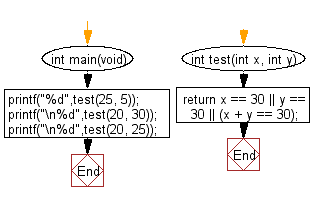﻿ C Program: 2 integers, one is 30 or their sum is 30# C Exercises: Check two given integers, and return true if one of them is 30 or if their sum is 30

## C-programming basic algorithm: Exercise-3 with Solution

Write a C program to check two given integers, and return true if one of them is 30 or if their sum is 30.

C Code:

``````#include <stdio.h>
int main(void){
printf("%d",test(25, 5));
printf("\n%d",test(20, 30));
printf("\n%d",test(20, 25));
}
int test(int x, int y)
{
return x == 30 || y == 30 || (x + y == 30);
}
``````

Sample Output:

```1
1
0
```

Pictorial Presentation:Flowchart:C Programming Code Editor:

What is the difficulty level of this exercise?

Test your Programming skills with w3resource's quiz.

﻿

## C Programming: Tips of the Day

How does array  = {0} set the entire array to 0?

The behavior of this code in C is described in section 6.7.8.21 of the C specification (online draft of C spec): for the elements that don't have a specified value, the compiler initializes pointers to NULL and arithmetic types to zero (and recursively applies this to aggregates).

The behavior of this code in C++ is described in section 8.5.1.7 of the C++ specification (online draft of C++ spec): the compiler aggregate-initializes the elements that don't have a specified value.

Also, note that in C++ (but not C), you can use an empty initializer list, causing the compiler to aggregate-initialize all of the elements of the array:

`char array = {};`

As for what sort of code the compiler might generate when you do this, take a look at this question: Strange assembly from array 0-initialization

Ref : https://bit.ly/3yxvK5c